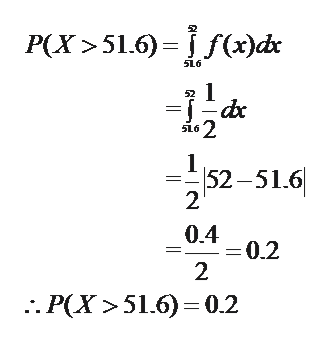# The lengths of a professor's classes have a continuous uniform distribution between 50.0 min and 52.0 min. If one such class is randomly selected, find the probability that the class length is more than 51.6 min.P(X > 51.6) = (Report answer accurate to 2 decimal places.)

Question
243 views
The lengths of a professor's classes have a continuous uniform distribution between 50.0 min and 52.0 min. If one such class is randomly selected, find the probability that the class length is more than 51.6 min.

P(X > 51.6) =
(Report answer accurate to 2 decimal places.)

check_circle

Step 1

Solution: We are given that the lengths of a professor's classes have a continuous uniform distribution between 50.0 min and 52.0 min.

Therefore, the probability density function of the given random variale is:

Step 2

We have to find the probability that the class length is more than 51.6 min....help_outlineImage TranscriptioncloseР(X >51.6) - Fjd SL6 1 52-51.6 2 0.4 =0.2 2 :. Р(Х >51.6) - 0.2 fullscreen

### Want to see the full answer?

See Solution

#### Want to see this answer and more?

Solutions are written by subject experts who are available 24/7. Questions are typically answered within 1 hour.*

See Solution
*Response times may vary by subject and question.
Tagged in

### Measures